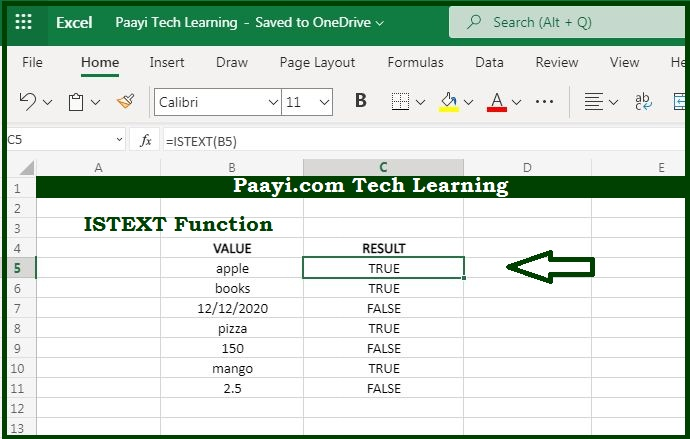# Learn How to Use Microsoft Excel ISTEXT Function

Written by | 0 Comments | 695 Views

In this article, you will learn how to use the Microsoft Excel ISTEXT function and its prime function in Microsoft Excel. You will also get to know the Microsoft Excel ISTEXT function return value and syntax with the help of some examples.

Microsoft Excel ISTEXT Function

The main function of the Microsoft Excel ISTEXT function is to test for the text value. That means with the help of ISTEXT function you can able to get the return value as the TRUE if the cell contains text, and FALSE if it not. You can also use the ISTEXT function to check whether the cell contains the text value or a numeric value entered as a text.

Return Value of ISTEXT Function

The return value will be logical TRUE or FALSE.

Syntax of ISTEXT Function

=ISTEXT(value)

Where the arguments:

value: It is the value to check.

## How to Use Microsoft Excel ISTEXT Function?The main function of the Microsoft Excel ISTEXT function is to test for a text. That means with the help of ISTEXT function you can able to get the return value as the TRUE if the cell contains text, and FALSE if it does not

It should be noted that:

Usually, the value is supplied as a cell address.

The ISTEXT function is the part of the "IS" function that returns the logical value TRUE or FALSE.